# 3-3: You Can Handle The Proof

In this lesson we describe how to make theorem-like environments and proofs, and how to refer back to theorems, figures, sections, and tables with automatic numbering (the \label{} and \ref{} commands).

## The Proof is in the Pudding

The amsthm package is the most common mechanism for inserting theorems, corollaries, and proofs into your LaTeX document. One you’ve included it, writing a proof environment like

\begin{proof}
Here is some text for the proof.
\end{proof}

will produce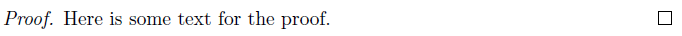The box at the bottom-right hand corner of the proof is used to help clarify for the reader where a proof ends (it’s especially helpful when you have long or nested proofs, or want to skip reading it for now). It is called a Halmos (or tombstone), named after the Hungarian mathematician Paul Halmos, who was renowned for his expository skills.

Now, what shall we prove? Typically, mathematicians call the different results constituting a document as follows:

• a theorem is a main results of a paper
• a lemma is a result that might not be of obvious importance, except that you will use it to prove something else
• a corollary is a small secondary result that follows relatively easily from another result
• claim, observation or fact means a smaller or easier result, usually to emphasize the structure of a larger proof or just to be stated for future reference
• conjecture is a statement which the author thinks is true, but has not proved.

You also often will see definitions, questions, remarks, and examples. All of these are called theorem-like environments when you use amsthm. The first step in using one is to define it with a newtheorem command. For example

\newtheorem{theorem}{Theorem}

will define a new theorem environment, which will automatically be labeled with numbers like Theorem 1, Theorem 2, etc. Read the following source code, which contains additional information.

\newtheorem{theorem}{Theorem}              % typically in the preamble
\newtheorem{corollary}[theorem]{Corollary} % typically in the preamble
% the [theorem] in the definition of corollary tells amsthm that you
% don't want separate numbering for corollaries, but instead to use the
% same automatically-generated counter as for theorems.
\begin{theorem}
Theorems are awesome.
\end{theorem}
\begin{corollary}[The contrapositive]      % [nickname, year, or author]
Non-awesome things are not theorems.
\end{corollary}

This produces: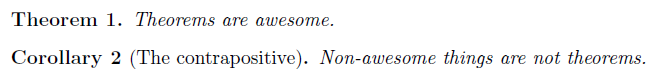Normally, you would follow each theorem-like environment with a proof.

For a definition environment, the traditional style is typically different (for example, not in italics). To enforce this in amsthm, use the \theoremstyle{definition} command: all of the theorem-like environment definitions appearing after this command will be written in that way.

For more information on styles and numbering, check out the amsthm documentation. For example, you can suppress a number in a theorem’s name, or have the theorems numbered by section and then sub-numbered.

### \qedhere

Occasionally you will want to end a proof with an equation; it’s generally not a recommended style but does have its place. Ending the proof like $$f(x)=y$$\end{proof} causes things to look bad: the halmos will appear on a blank line by itself, like: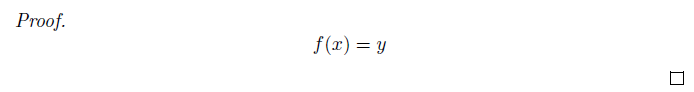To fix this, you should ensure amsmath is included, and you should change the end of the proof to

\begin{equation}       % instead of $f(x)=y\tag*{\qedhere} % the \tag*{} is new \end{equation} % instead of$
\end{proof}

This produces the halmos in the right place: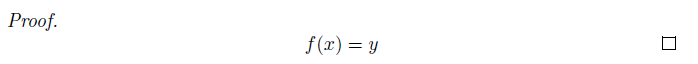Here is an optional explanation for the curious: we use an equation environment so that a number can appear; the \tag{symbol} command lets you put a special symbol as the “number” for an equation, instead of the automatically-generated number, and is part of amsmath; \tag* is like \tag but does not show the parentheses; and \qedhere prints the halmos immediately, while also suppressing it from appearing later on.

## Referencing Numbers

One advantage of LaTeX is that is automatically generates numbers for theorems, sections, figures, tables, et cetera. What’s even cooler is that you can refer back to these theorems while producing the automatically-generated numbers correctly. In the text, this would appear as “By inequality (5)…” or “As you can see in Figure 2…” or “As we mentioned in the proof of Theorem 3…”

There are two steps:

• use \label{label-name} immediately after the start of the theorem-like environment, figure caption, equation definition, or (sub)section title, to create a new label in LaTeX that will hold this number
• use \ref{label-name} to automatically print the same number. You can also use \eqref{label-name} to get the same number (requires amsmath) surrounded by parentheses, which is normally how equations are referenced.

For example,

In Section \ref{sec:greece} we'll discuss some historical theorems.
\section{Great Theorems of Ancient Greece} \label{sec:greece}
\begin{equation} a^2+b^2=c^2 \label{eq:pythagorus} \end{equation}
Equation \eqref{eq:pythagorus} was proven by Pythagorus.

creates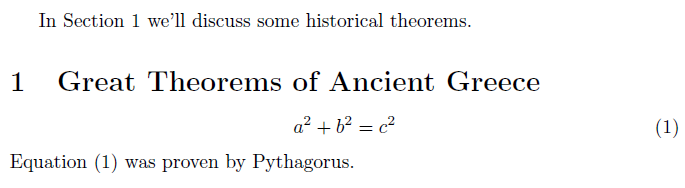Here are a few remarks:

• Label an equation like this:
\begin{equation}a^2+b^2=c^2\label{eq:pythag}\end{equation}
• For multi-line equations like align, move the \label{} so that there are no \\ (newlines) between the \label{} and the particular line you want to refer to.
• Label a caption, if you want to refer to a figure, table, or other float:
\caption{The caption.\label{fig:photo}}
• Label items in enumerated lists like this:
\item \label{item:warn} Watch out!
• We recommend using consistent label names like eq:whatever and sec:whatever, but it is not required.
• If you are running LaTeX at home, you may need to run the file two times or more for the references to show up correctly. (This has to do with the .aux files that LaTeX generates.)
• If you are using the hyperref package, then all \ref{} commands will produce clickable links that jump you to the item being referenced.
• The prettyref package allows you to do additional things like automatically prepending the item type (such as Theorem).
• \pageref{label} will give you the number of the page on which the given label appears.

### Why do I need to make labels? Can’t I just use the numbers?

It seems that referencing is a little bit of a convoluted method.  You label in order to reference an environment, then reference it to get the number the environment was labelled.  Why bother?  The reason for this is that it greatly simplifies any editing that needs to be done.

If you ever needed to:

• move a labelled environment elsewhere in the document,
• add an extra label somewhere in your document, or
• remove a labelled item from your document,

your entire numbering system could be thrown off by one or two numbers.  Because of the labeling system, you prevent having to go through your document and relabel every reference to items that have changed, since LaTeX knows the specific equation/environment/etc. you want to reference.

That’s all for this lesson!

These are course notes for the University of Waterloo's course Math 600: Mathematical Software.
© 2012—. Written and developed by David Pritchard and Stephen Tosh. Contact (goes to the CEMC)